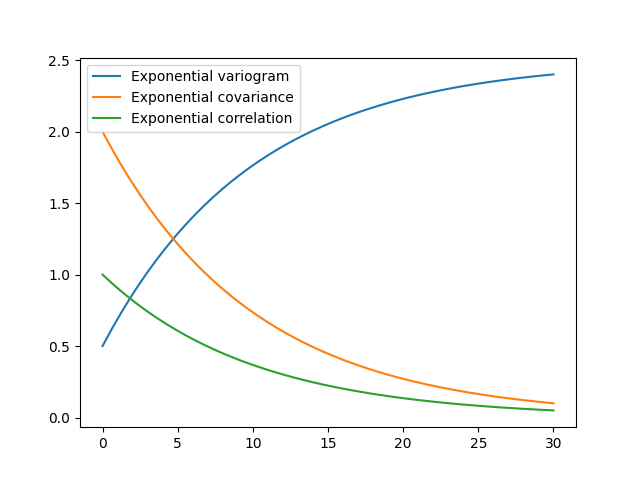# Basic Methods¶

The covariance model class CovModel of GSTools provides a set of handy methods.

One of the following functions defines the main characterization of the variogram:

• CovModel.variogram : The variogram of the model given by• CovModel.covariance : The (auto-)covariance of the model given by• CovModel.correlation : The (auto-)correlation (or normalized covariance) of the model given by• CovModel.cor : The normalized correlation taking a normalized range given by:As you can see, it is the easiest way to define a covariance model by giving a correlation function as demonstrated in the introductory example. If one of the above functions is given, the others will be determined:import gstools as gs

model = gs.Exponential(dim=3, var=2.0, len_scale=10, nugget=0.5)
ax = model.plot("variogram")
model.plot("covariance", ax=ax)
model.plot("correlation", ax=ax)


Total running time of the script: ( 0 minutes 0.118 seconds)

Gallery generated by Sphinx-Gallery